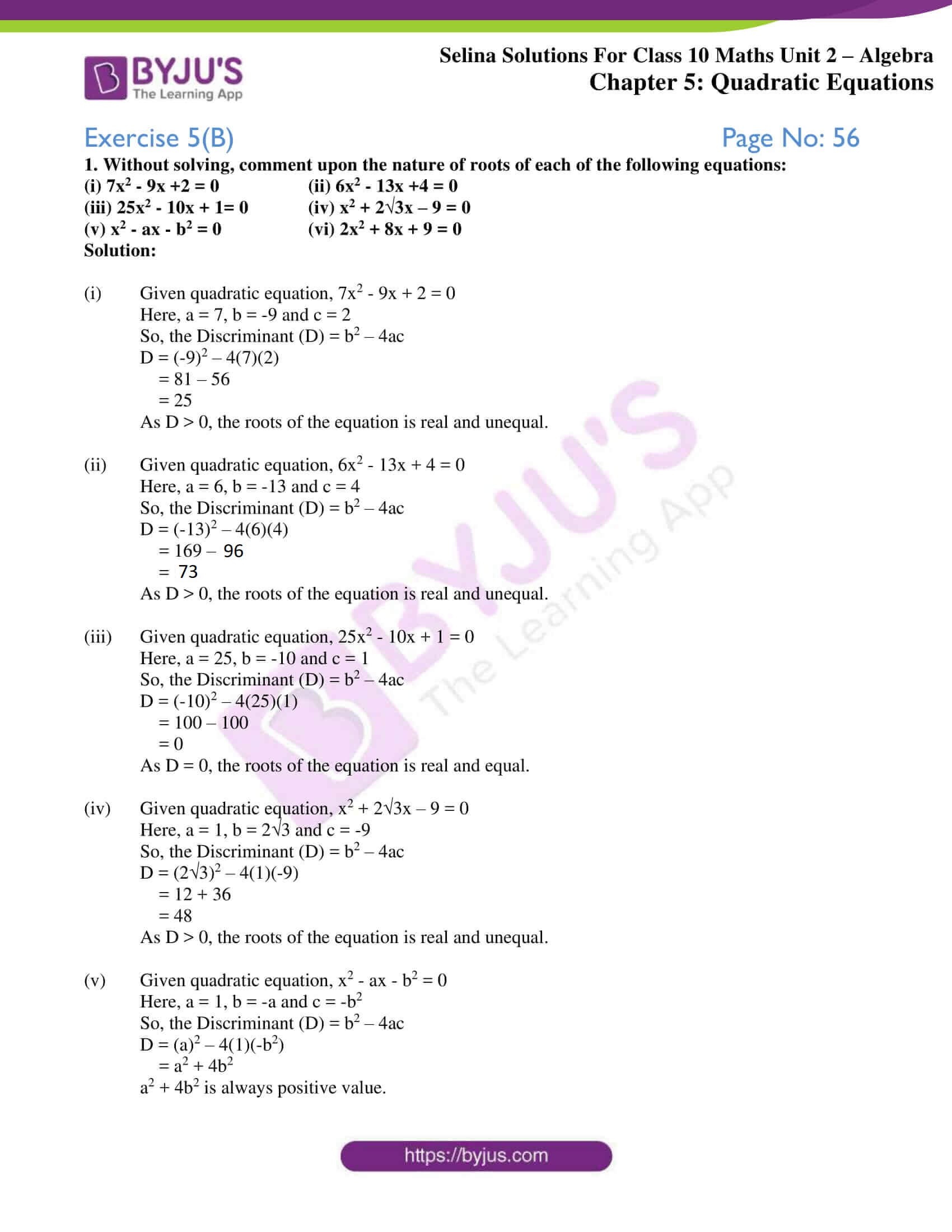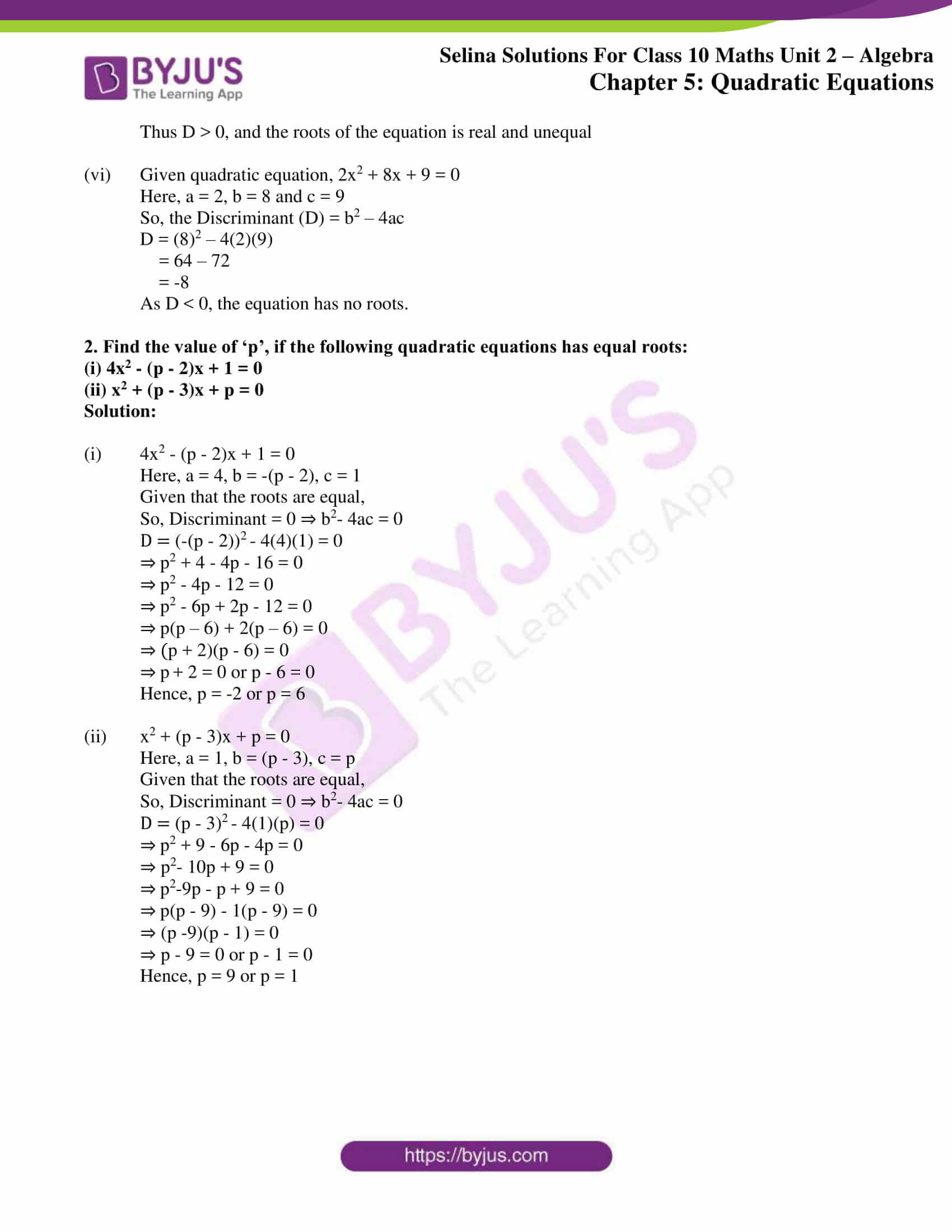# Selina Solutions Concise Maths Class 10 Chapter 5 Quadratic Equations Exercise 5(B)

A quadratic equation can have at most two roots. The nature of these roots is examined by finding the discriminant. Thus, this exercise has problems on finding the nature of roots of the given quadratic equation. For solutions to any exercise-wise problem of any chapter the Selina Solutions for Class 10 Maths can be utilized according to the student’s need. Additionally, the solutions of this exercise can be found in the Concise Selina Solutions for Class 10 Maths Chapter 5 Quadratic Equations Exercise 5(B) PDF provided in the links below.### Access other exercises of Selina Solutions Concise Maths Class 10 Chapter 5

Exercise 5(A) Solutions

Exercise 5(C) Solutions

Exercise 5(D) Solutions

Exercise 5(E) Solutions

Exercise 5(F) Solutions

### Access Selina Solutions Concise Maths Class 10 Chapter 5 Quadratic Equations Exercise 5(B)

1. Without solving, comment upon the nature of roots of each of the following equations:

(i) 7x2 – 9x +2 = 0 (ii) 6x2 – 13x +4 = 0

(iii) 25x2 – 10x + 1= 0 (iv) x2 + 2√3x – 9 = 0

(v) x2 – ax – b2 = 0 (vi) 2x2 + 8x + 9 = 0

Solution:

(i) Given quadratic equation, 7x2 – 9x + 2 = 0

Here, a = 7, b = -9 and c = 2

So, the Discriminant (D) = b2 – 4ac

D = (-9)2 – 4(7)(2)

= 81 – 56

= 25

As D > 0, the roots of the equation is real and unequal.

(ii) Given quadratic equation, 6x2 – 13x + 4 = 0

Here, a = 6, b = -13 and c = 4

So, the Discriminant (D) = b2 – 4ac

D = (-13)2 – 4(6)(4)

= 169 – 96

= 73

As D > 0, the roots of the equation is real and unequal.

(iii) Given quadratic equation, 25x2 – 10x + 1 = 0

Here, a = 25, b = -10 and c = 1

So, the Discriminant (D) = b2 – 4ac

D = (-10)2 – 4(25)(1)

= 100 – 100

= 0

As D = 0, the roots of the equation is real and equal.

(iv) Given quadratic equation, x2 + 2√3x – 9 = 0

Here, a = 1, b = 2√3 and c = -9

So, the Discriminant (D) = b2 – 4ac

D = (2√3)2 – 4(1)(-9)

= 12 + 36

= 48

As D > 0, the roots of the equation is real and unequal.

(v) Given quadratic equation, x2 – ax – b2 = 0

Here, a = 1, b = -a and c = -b2

So, the Discriminant (D) = b2 – 4ac

D = (a)2 – 4(1)(-b2)

= a2 + 4b2

a2 + 4b2 is always positive value.

Thus D > 0, and the roots of the equation is real and unequal

(vi) Given quadratic equation, 2x2 + 8x + 9 = 0

Here, a = 2, b = 8 and c = 9

So, the Discriminant (D) = b2 – 4ac

D = (8)2 – 4(2)(9)

= 64 – 72

= -8

As D < 0, the equation has no roots.

2. Find the value of ‘p’, if the following quadratic equations has equal roots:

(i) 4x2 – (p – 2)x + 1 = 0

(ii) x2 + (p – 3)x + p = 0

Solution:

(i) 4x2 – (p – 2)x + 1 = 0

Here, a = 4, b = -(p – 2), c = 1

Given that the roots are equal,

So, Discriminant = 0 ⇒ b2– 4ac = 0

D = (-(p – 2))2 – 4(4)(1) = 0

⇒ p2 + 4 – 4p – 16 = 0

⇒ p2 – 4p – 12 = 0

⇒ p2 – 6p + 2p – 12 = 0

⇒ p(p – 6) + 2(p – 6) = 0

⇒ (p + 2)(p – 6) = 0

⇒ p + 2 = 0 or p – 6 = 0

Hence, p = -2 or p = 6

(ii) x2 + (p – 3)x + p = 0

Here, a = 1, b = (p – 3), c = p

Given that the roots are equal,

So, Discriminant = 0 ⇒ b2– 4ac = 0

D = (p – 3)2 – 4(1)(p) = 0

⇒ p2 + 9 – 6p – 4p = 0

⇒ p2– 10p + 9 = 0

⇒ p2-9p – p + 9 = 0

⇒ p(p – 9) – 1(p – 9) = 0

⇒ (p -9)(p – 1) = 0

⇒ p – 9 = 0 or p – 1 = 0

Hence, p = 9 or p = 1